# HOMER Pro 3.12Note: With version 3.9, MATLAB Link works with both 32-bit and 64-bit MATLAB. With version 3.10, MATLAB Link users can pass additional variables to their dispatch.

The HOMER Pro MATLAB Link allows you to write your own dispatch algorithm for HOMER Pro using MATLAB. HOMER  interacts with the MATLAB software to run your MATLAB functions during the simulation. In order to run a simulation with your own MATLAB dispatch algorithm you need to have:

HOMER Pro 3.7 or later installed

32-bit/64-bit version of MATLAB installed and licensed

Three MATLAB functions described below (these comprise your custom algorithm; example M-files are included below)

A HOMER Pro MATLAB Link Controller created on the Controller Set Up page, with the settings described below

## MATLAB Functions

You need three MATLAB functions, each in a separate M-file. Place the three M-files together in a directory. Specify the location and name of these files in HOMER on the Controller page so that it knows how to run your functions. The functions must use the following syntax exactly:

[myErr, custom_variables] = MatlabStartSimulation(simulation_parameters)

[simulation_state, custom_variables] = MatlabDispatch(simulation_parameters, simulation_state, custom_variables)

myErrs = MatlabEndSimulation(simulation_parameters, custom_variables)

HOMER calls these three commands before, during, and after the simulation. To run a simulation with the MATLAB Link, HOMER performs the following steps:

1.The HOMER Pro MATLAB Link Controller opens an instance of MATLAB and sends the simulation_parameters variable to the MATLAB workspace.

2.HOMER runs the MatlabStartSimulation command in MATLAB.

3.HOMER gets the myErr variable back from MATLAB. If myErr contains an error, HOMER may stop the simulation or the entire calculation as described in MatlabStartSimulation.

4.The custom_variables variable is left in the MATLAB workspace so it is accessible by all the following MATLAB function calls.

5.HOMER creates the simulation_state variable and sends it to MATLAB.

6.HOMER runs the command for MatlabDispatch in MATLAB.

7.HOMER reads the new values set by MatlabDispatch in the simulation_state variable and simulates the time step according to these dispatch commands.

8.HOMER updates the values in simulation_state for the new time step. Steps 6, 7, and 8 are repeated until all the time steps are simulated.

9.Finally, after all the time steps are simulated, HOMER calls MatlabEndSimulation. Any errors are reported in the HOMER user interface.

While the simulation_parameters variable is not changed throughout the simulation, the values in the simulation_state variable are changed in each time step to reflect how the system is operating. For each time step, the simulation_state variable is updated in the MATLAB workspace, and the MatlabDispatch function is called. The MatlabDispatch function makes changes to the simulation_state variable, which are read by HOMER for each time step.

Each MATLAB function must follow the syntax given above. The topics below have more details and examples for each function.

## Setting Up the HOMER Model

To run you MATLAB dispatch code, you must set up the MATLAB Link Controller for your model. On the Controller Set Up page, select HOMER Pro MATLAB Link from the drop-down menu. Click the Add button. HOMER adds a new tab for the HOMER Pro MATLAB Link and opens that Controller page with the following variables and defaults.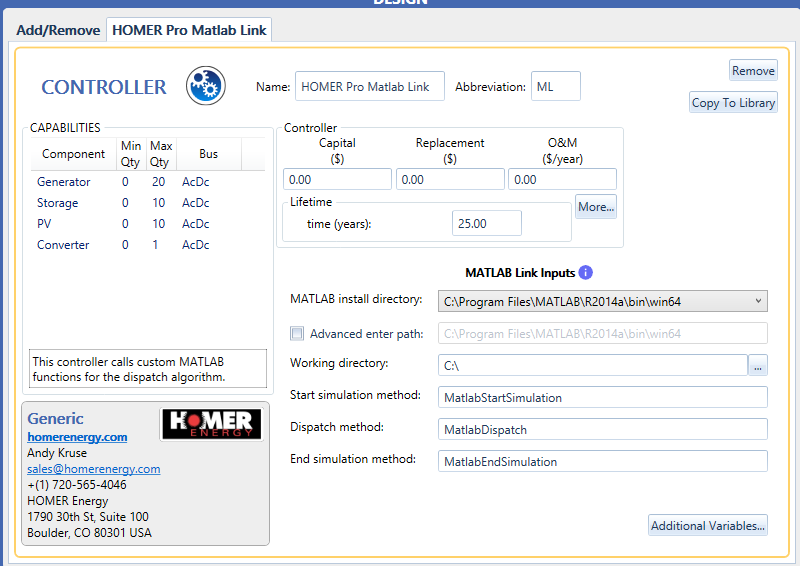The following table provides descriptions of these options.

 Variable Description Path to '\bin\win32' (if you are using 32 bit version Path to '\bin\win64' (if you are using the 64 bit version) subfolder in MATLAB installation directory Enter the path to the installation of MATLAB on your computer, to the '\bin\win32' '\bin\win64' subfolder there. HOMER needs the 32-bit/64-bit installation of MATLAB. (You can have 32-bit and a 64-bit versions of MATLAB installed on your computer at the same time.) A typical path is: C:\Program Files (x86)\MATLAB\R2015b\bin\win32 Working directory The location of the M-files with the three functions defined above. HOMER sets this as the MATLAB working directory, and calls the functions as described above. Start simulation method The name for your MatlabStartSimulation function that is called before the simulation. In related help sections, the function is called MatlabStartSimulation, but you can call it whatever you want, and specify that name here. Dispatch method The name for the MatlabDispatch function that is called for each time step. In related help sections, this function is called MatlabDispatch, but you can name it something else if desired. End simulation method The name for your MatlabEndSimulation function that is called after the simulation. In related help sections, this function is called MatlabEndSimulation, but you can choose a different name.

With 3.10, you can use additional variables. You can even define the type (Number, String, or Boolean)

Step 1: On the MATLAB Link Controller page, click on the Additional Variables button. A pop-up window opens allowing you to use the green plus button to add new variables.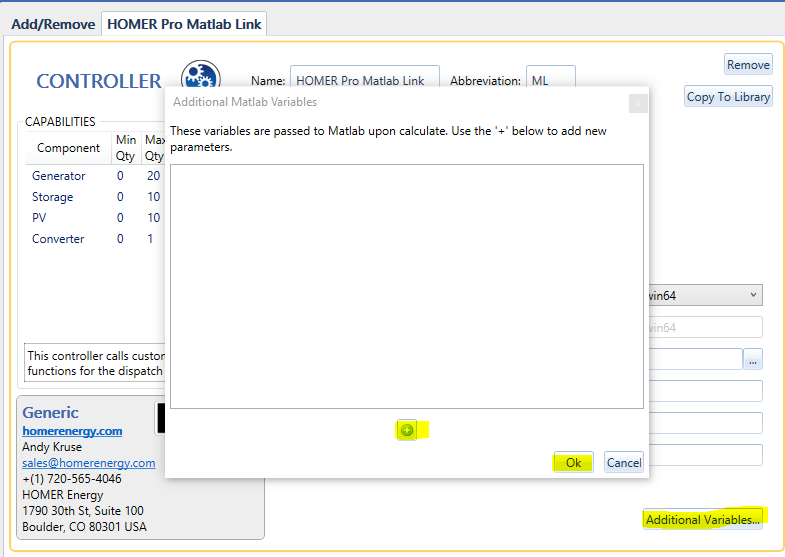Step 2: Choose the type of the new variable (Number, String, or Check-box). Enter the name and description of the variable.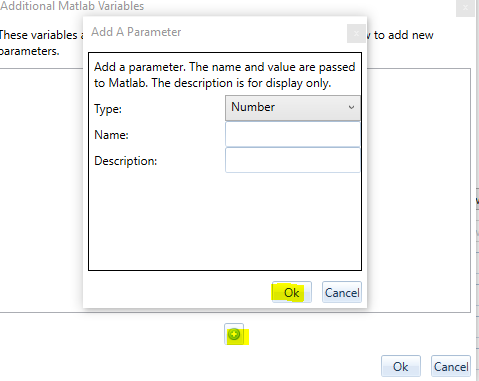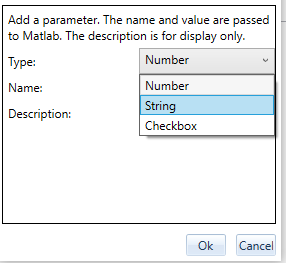Step 3: Click OK to save the additional variable.

## Troubleshooting

If errors occur while running the MATLAB dispatch, you may see the message "No feasible solutions found."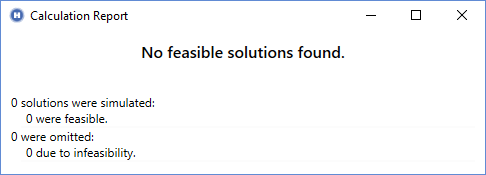First, ensure that your system actually produces at least one feasible result by running your system with one of the original HOMER dispatch algorithms, Cycle Charging or Load Following.

Look in the message list in the lower-left corner of the HOMER page for specific error messages. You can hover your mouse over a message or click on it to see the full message text. One potential issue is that HOMER might be unable to find your MATLAB installation. If this occurs, the DLL Load Library Failed error message appears.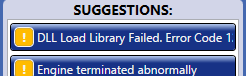There are three common causes for this error message:

The "Path to the MATLAB installation directory" input is incorrect.

The "Path to the MATLAB installation directory" points to a 32-bit/64-bit version of MATLAB and you are using 64-bit HOMER Pro, or it points to a 64-bit version of MATLAB and you are using 32-bit HOMER Pro.

If you see this message, ensure that the path specified for the input "Path to the MATLAB installation directory" is correct. Also check that you are accessing the correct version of MATLAB (32-bit or 64-bit) to match your version of HOMER Pro. If you are using the 64-bit version of HOMER Pro, you'll see the letters "x64" in the HOMER Pro title bar. On most 64-bit computers, 32-bit programs are installed in "Program Files (x86)" and not in "Program Files". If you need a different version of MATLAB, you can download it from the MathWorks website. You can have both 32-bit and 64-bit versions of both MATLAB and HOMER Pro installed on your computer at the same time.

Make sure you can run MATLAB outside of HOMER Pro. If you do not have an active MATLAB license, the MATLAB Link does not work.

### "Cannot start Matlab Engine: code -3"

If HOMER is able to find your MATLAB installation, but it cannot open MATLAB. One possible cause of this error is that your installation of MATLAB is not registered with your operating system. To fix it, open a command window as administrator (right-click on the Command window icon and choose "run as administrator." Enter the command "matlab /regserver" to tell MATLAB to re-register itself on your computer.

### "Error Using cd"

If HOMER is able to find your MATLAB installation, but not the working directory you specified, the "MATLAB error: Error using cd..." error message appears in the message list in the lower-left corner of the HOMER Pro window.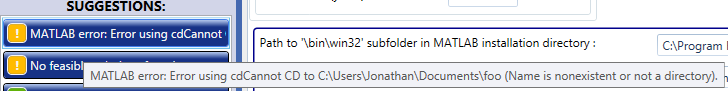Hover your mouse over the message to see the full text. If you see this error, check the path you specified for the "Working directory" entry. The path might be spelled wrong or not exist. HOMER gives MATLAB the cd('C:\your\path') command where "C:\your\path" is the path you specified for the "Working directory" entry, and MATLAB gives an error. If it is not clear by checking the path name, open MATLAB and use the "cd" command to debug this step.

### MATLAB Error While Executing

When the steps above are completed, HOMER sends the simulation_parameters variable to the MATLAB workspace, and then calls your MatlabStartSimulation command using the syntax listed above. If any of the three functions are missing, or if the names are spelled wrong, HOMER provides the corresponding error message in the Suggestions area in the lower-left area of the page when the calculation is finished.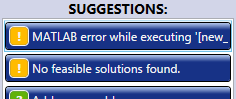You can hover your mouse over the message or click it to see the full text of the error message. In this example, the full text of the message is:

MATLAB error while executing '[new_simulation_state, custom_variables] = MatlabDispatchWrong(simulation_parameters, simulation_state, custom_variables);': Undefined function 'MatlabDispatchWrong for input arguments of type 'struct'.

This message can be broken down into several parts to make it easier to understand. The first part of the message says: MATLAB error while executing 'stuff':. The stuff in quotes is the exact command that HOMER gives to MATLAB. In this example, it is:

[new_simulation_state, custom_variables] = MatlabDispatchWrong(simulation_parameters, simulation_state, custom_variables);

Verify that this command is correct. The second part of the error message is the exact message from MATLAB:

Undefined function 'MatlabDispatchWrong' for input arguments of type 'struct'.

This as MATLAB's way of stating that it could not find the MatlabDispatchWrong function. The error message provides information about what went wrong.

Another issue is that your M-file might cause an error while it is running. If that happens, the error message is similar to the example above, except the second part of the message, which contains MATLAB's exact error message, is different. It states what went wrong and may give you the number of the line in your M-file where the error occurred. For example:

MATLAB error while executing '[new_simulation_state, custom_variables] = MatlabDispatch(simulation_parameters, simulation_state, custom_variables);': Reference to non-existent field 'wrong_field_name'. Error in MatlabDispatch (line 30) simulation_state.wrong_field_name;

You can see from the error message that the MatlabDispatch function tried to access a field of the simulation_state structure that does not exist, and it happened on line 30 of that function (which is written in the MatlabDispatch.m file).

### Debugging Interactively

Sometimes the error message alone can be enough to find and fix a mistake in the MATLAB code, but other times it helps to use the MATLAB IDE to interactively debug the dispatch code. In the function that you want to debug, add a line of code to save the workspace:

save('testvariables.mat');

You can add this command anywhere in the MatlabStartSimulation, MatlabDispatch, or MatlabEndSimulation functions. If you add it to the MatlabDispatch function, you might want to use a condition so it only performs the save once, and not again for each time step:

if simulation_state.current_timestep == 1

save('testvariables.mat');

end

After you make this modification, open your model (with the MATLAB Link Controller) in HOMER and click the Calculate button in the toolbar to run the MATLAB dispatch. This runs your MATLAB functions and saves the .mat file. If you entered a full path in the save('...') command, MATLAB saves the .mat file in the location specified. If you specified a file name with no path or with a relative path, MATLAB saves the .mat file in the current working directory (or evaluates the relative path with respect to the working directory) that you specified in the Controller component.

Open MATLAB and set the working directory to the location of your dispatch M-files. When you load the saved .mat file, the variables that were in your dispatch function appear in your workspace. For example, if you put the save command in the MatlabDispatch function; simulation_parameters, simulation_state, and any other working variables created by the MatlabDispatch function, appear in your workspace. If you put the save('...') command in MatlabStartSimulation or MatlabEndSimulation, simulation_parameters are included in the .mat file, but the simulation_state is not, because it is not present when those functions are executing.

With MATLAB open, and the saved .mat file loaded, you can set a breakpoint in your function, run it from the command window, and debug it interactively. See the Listing of simulation_state and Listing of simulation_parameters sections in the help for more information about these variables.